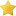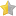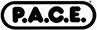# Descriptive Statistics (Online CE Course)(based on 236 customer ratings)

How to Subscribe
 MLS & MLT Comprehensive CE PackageIncludes 173 CE courses, most popular \$95 Pick Your CoursesUp to 8 CE hours \$50 Individual course \$20
Need multiple seats for your university or lab? Get a quote

Statistics is the branch of mathematics that deals with the organization, analysis, and interpretation of information. This course discusses statistics that are used in the laboratory for assessment of quality control. In this course you will primarily learn about descriptive statistics which deals with the enumeration, organization, and graphic representation of data.
This course is part of a series of courses adapted for the web by MediaLab Inc. under license from Educational Materials for Health Professionals Inc. Dayton OH, 45420. Copyright EMHP.

See all available courses

### Continuing Education CreditsP.A.C.E.® Contact Hours (acceptable for AMT, ASCP, and state recertification): 2 hour(s)Approved through 7/31/2024Florida Board of Clinical Laboratory Personnel Credit Hours - General: 2 hour(s)Approved through 7/31/2024

### Objectives

• Explain why knowledge of statistics is important to laboratory scientists.
• Compare and contrast the following terms:
• sample and population
• data, statistic, and parameter
• independent and dependent variable
• discrete and continuous data
• Prepare tables and figures of data that meet the criteria specified in this unit.
• Describe "frequency distribution" and explain the advantage of presenting data in this manner.
• Given a set of data, construct a frequency table according to criteria stated in this unit.
• Given a set of data, construct both actual and relative frequency distributions that accurately represent the discrete or continuous nature of the data.
• Define and calculate the following measures of central tendency:
• mean
• median
• mode
• Learn what percentage of data, in a normal distribution, is encompassed by 1, 2, and 3 standard deviations.
• Given a set of data, calculate its variance and standard deviation.
• Given the mean and standard deviation of a set of data in a normal distribution, find the ranges encompassing 68%, 95%, and 99% of all observations.
• Describe at least two situations in which coefficient of variation is a useful tool.
• Calculate coefficient of variation when given the mean and standard deviation of a set of data.
• Explain how coefficient of variation is used in the clinical laboratory.
• Upon completion of this course, the student will be able to use the appropriate descriptive statistics principles to present and analyze data.

### Customer Ratings(based on 236 customer ratings)

### Course Outline

Click on the links below to preview selected pages from this course.
• Introduction to Statistics
• Introduction to Statistics
• Why Statistics?
• Descriptive Statistics
• What common tasks in the clinical laboratory require knowledge of basic statistics?
• Definition of Statistics Terms
• Samples and Populations
• Data, Statistics, and Parameters
• Independent and Dependent Variables
• Discrete and Continuous Data
• Read the following passage, and identify the underlined words: The researchers tested 50 students chosen at random who had taken calculus in high scho...
• Read the following passage, and identify the underlined words: The researchers tested 50 students chosen at random who had taken calculus in high scho...
• Read the following passage, and identify the underlined words: The researchers tested 50 students chosen at random who had taken calculus in high scho...
• Identify whether the underlined phrase is an independent or dependent variable: The amount of sunlight that plants receive is directly proportional to...
• Graphic Representation of Data
• Importance of Professional Tables and Graphs
• Table Specifications
• Figures Specification
• What is wrong with the following table? Plant #HeightSunlight112621593834251252915
• What is wrong with the following bar chart?Figure 3
• Frequency Distribution
• Data and Data Pairs
• Using Frequency Distributions
• Constructing Frequency Distributions
• A Frequency Distribution Example
• Step 1: Select Size and Number of Class Intervals
• Step 2: List the Class Intervals
• Step 3: Sort the Data
• Step 4: Determine Frequencies
• Step 5: Determine Relative Frequencies
• Graphing Frequency Distributions
• Bar Chart
• Histogram
• Frequency Polygon
• Absolute vs. Relative Frequency
• Use the following data for the next four questions: Table 5: Serum BUN values (mg/dL) from hospital employees at Kettering Medical Center15.922.59.116...
• Table 5: Serum BUN values (mg/dL) from hospital employees at Kettering Medical Center 15.922.59.116.86.428.615.223.710.317.013.820.57.425.04.118.412.7...
• Table 5: Serum BUN values (mg/dL) from hospital employees at Kettering Medical Center 15.922.59.116.86.428.615.223.710.317.013.820.57.425.04.118.412.7...
• Table 5: Serum BUN values (mg/dL) from hospital employees at Kettering Medical Center 15.922.59.116.86.428.615.223.710.317.013.820.57.425.04.118.412.7...
• Measures of Central Tendency
• Mean, Median, and Mode
• Mean
• Median
• Mode
• Use the following data for the next three questions: Table 6: Number of children in household for 20 households in Atlanta, Georgia 424112150310231216...
• Table 6: Number of children in household for 20 households in Atlanta, Georgia 42411215031023121610 What is the median number of children in a househo...
• Table 6: Number of children in household for 20 households in Atlanta, Georgia 42411215031023121610 What is the mode of the number of children in a ho...
• Standard Deviation
• Standard Deviation (continued)
• Standard Deviation Example
• Standard Deviation Example (continued)
• Use the data for the following question: Table 7: Urea Nitrogen Concentration in 9 Employees (mg/dL) Concentration (mg/dL)x-(x-)210111113951579Total W...
• Normal Distribution
• Introduction to the Normal Distribution
• Normal Distribution
• 68-95-99 Rule Illustrated
• Inferences from Sample Data
• Suppose you measured the Serum BUN levels in a sample of several healthy people. You found that the average was 19.6 mg/dL and the standard deviation ...
• Your supervisor asks you to give the 95% range of normal Serum BUN levels, the range within which 95% of healthy people will fall. What is this range?...
• Coefficient of Variation
• A Measure of Relative Variability
• Using the Coefficient of Variation
• Monitoring Methods
• What is the coefficient of variation of the data you used in the previous section? ( = 19.6 mg/dL, s = 6.1 mg/dL)
• References
• References